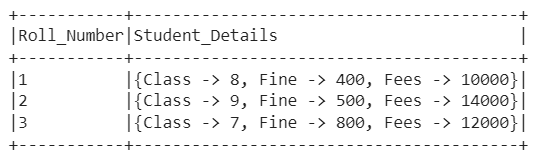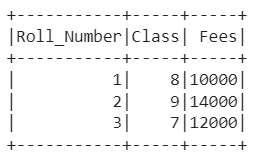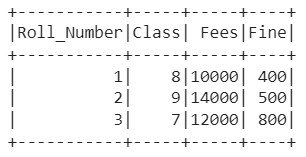Open in App
Not now

# Converting a PySpark Map/Dictionary to Multiple Columns

• Last Updated : 23 Jan, 2023

In this article, we are going to learn about converting a column of type ‘map’ to multiple columns in a data frame using Pyspark in Python.

A data type that represents Python Dictionary to store key-value pair, a MapType object and comprises three fields, keyType, valueType, and valueContainsNull is called map type in Pyspark. There may occur some situations in which we get data in the form of a map in the Pyspark data frame column, but the user wants them in the different columns for applying functions on those columns. This can be achieved in Pyspark easily not only in one way but through numerous ways which are explained in this article.

### Methods to convert a column of type ‘map’ to multiple columns in a Pyspark data frame:

• Using withColumn() function
• Using list and map() functions
• Using explode() function

### Method 1: Using withColumn() function

A transformation function of a data frame that is used to change the value, convert the datatype of an existing column, and create a new column is known as withColumn() function. In this method, we will see how we can convert a column of type ‘map’ to multiple columns in a data frame using withColumn() function. What we will do is use withColumn() function with a new column name and map key as arguments.

Syntax: df.withColumn(“new_column_name”, col(“mapped_column”)[“mapkey_name”])

Parameters:

• mapped_column: It is the column which is mapped and has various map keys in it.
• mapkey_name: It is the values of map key which will be used to create new columns.
• new_column_name: It is the name of the new column that has to be formed.

Example:

In this example, we have created the data frame with two columns ‘Roll_Number‘ and ‘Student_Details‘. The ‘Student_Details‘ is a map-type column that has Class, Fine, and Fees as map keys as follows:Once the data frame is created, we created new columns in the data frame for Class and Fees using withColumn() function with a new column name and particular map key as arguments. Finally, we displayed the data frame.

## Python3

 `# Python program to convert a column of ` `# type 'map' to multiple columns ` `# in a Pyspark data frame using withColumn function ` ` `  `# Import the SparkSession and col libraries ` `from` `pyspark.sql ``import` `SparkSession ` `from` `pyspark.sql.functions ``import` `col ` ` `  `# Create a spark session ` `spark_session ``=` `SparkSession.builder.getOrCreate() ` ` `  `# Create a data frame with a map column type 'Student_Details' ` `df ``=` `spark_session.createDataFrame( ` `    ``[(``1``, {``"Class"``: ``8``, ``"Fees"``: ``10000``, ``"Fine"``: ``400``}), ` `     ``(``2``, {``"Class"``: ``9``, ``"Fees"``: ``14000``, ``"Fine"``: ``500``}), ` `        ``(``3``, {``"Class"``: ``7``, ``"Fees"``: ``12000``, ``"Fine"``: ``800``})], ` `    ``[``'Roll_Number'``, ``'Student_Details'``]) ` ` `  `# Convert map column to multiple columns 'Class' and 'Fees' ` `df ``=` `df.withColumn(``"Class"``, ` `                   ``col(``"Student_Details"``)[``"Class"``]).withColumn( ` `    ``"Fees"``, col(``"Student_Details"``)[``"Fees"``]) ` ` `  `# Drop the map column and display data frame ` `df.drop(``'Student_Details'``).show() `

Output:### Methods 2: Using list and map functions

A data structure in Python that is used to store single or multiple items is known as a list, while RDD transformation which is used to apply the transformation function on every element of the data frame is known as a map. In this method, we will see how we can convert a column of type ‘map’ to multiple columns in a data frame using list and map functions. What we will do is use the list() function with the mapped column along with map() function for mapping and map keys as arguments.

Example:

In this example, we have created the data frame with two columns ‘Roll_Number‘ and ‘Student_Details‘. The ‘Student_Details‘ is a map-type column that has Class, Fine, and Fees as map keys as follows:Once the data frame is created, we created new columns in the data frame for Class, Fees, and Fine using the list and map() function with mapped column and map keys as arguments. Finally, we displayed the data frame.

## Python3

 `# Python program to convert a column of type 'map' to multiple columns ` `# in a Pyspark data frame using list and map functions ` ` `  `# Import the SparkSession and col libraries ` `from` `pyspark.sql ``import` `SparkSession ` `from` `pyspark.sql.functions ``import` `col ` ` `  `# Create a spark session ` `spark_session ``=` `SparkSession.builder.getOrCreate() ` ` `  `# Create a data frame with a map column type 'Student_Details' ` `df ``=` `spark_session.createDataFrame([ ` `    ``(``1``, {``"Class"``: ``8``, ``"Fees"``: ``10000``, ``"Fine"``: ``400``}), ` `    ``(``2``, {``"Class"``: ``9``, ``"Fees"``: ``14000``, ``"Fine"``: ``500``}), ` `    ``(``3``, {``"Class"``: ``7``, ``"Fees"``: ``12000``, ``"Fine"``: ``800``})], ` `    ``[``'Roll_Number'``, ``'Student_Details'``]) ` ` `  `# Convert map column to multiple columns 'Class,' 'Fees' and 'Fine' ` `cols ``=` `[col(``"Roll_Number"``)] ``+` `list``( ` `    ``map``(``lambda` `f: col( ` `        ``"Student_Details"``).getItem(f).alias(``str``(f)), ` `        ``[``"Class"``, ``"Fees"``, ``"Fine"``])) ` ` `  `# Display the data frame ` `df.select(cols).show() `

Output:### Method 3: Using explode() function

The function that is used to explode or create array or map columns to rows is known as explode() function. In this method, we will see how we can convert a column of type ‘map’ to multiple columns in a data frame using explode function. What we will do is store column names of the data frame in a new data frame column by using explode() function and then transform that column into a list. Further, we get the value of each column from the data frame and display it.

Example:

In this example, we have created the data frame with two columns ‘Roll_Number‘ and ‘Student_Details‘. The ‘Student_Details‘ is a map-type column that has Class, Fine, and Fees as map keys as follows:Once the data frame is created, we exploded the data frame using explode function and further converted it into a list using rdd.map() function. Finally, we get the value for each column by using the list and map function from the data frame and displayed it.

## Python3

 `# Python program to convert a column of type 'map' to multiple columns  ` `# in a Pyspark data frame using explode function ` ` `  `# Import the SparkSession, explode, map_keys and col libraries ` `from` `pyspark.sql ``import` `SparkSession ` `from` `pyspark.sql.functions ``import` `col, explode, map_keys ` ` `  `# Create a spark session ` `spark_session ``=` `SparkSession.builder.getOrCreate() ` ` `  `# Create a data frame with a map column type 'Student_Details' ` `df``=``spark_session.createDataFrame( ` `  ``[(``1``, {``"Class"``:``8``, ``"Fees"``:``10000``, ``"Fine"``:``400``}), ` `   ``(``2``, {``"Class"``:``9``, ``"Fees"``:``14000``, ``"Fine"``:``500``}), ` `   ``(``3``, {``"Class"``:``7``, ``"Fees"``:``12000``, ``"Fine"``:``800``})], ` `  ``[``'Roll_Number'``, ``'Student_Details'``]) ` ` `  `# Store all the data frame columns in a new data frame column ` `exploded_df ``=` `df.select( ` `  ``explode(map_keys(df.Student_Details))).distinct() ` ` `  `# Convert exploded data frame column into the list ` `exploded_list ``=` `exploded_df.rdd.``map``( ` `                   ``lambda` `x:x[``0``]).collect() ` ` `  `# Get value for each column from the data frame ` `exploded_columns ``=` `list``( ` `  ``map``(``lambda` `x: col( ` `    ``"Student_Details"``).getItem(x).alias(``str``(x)), ` `      ``exploded_list)) ` ` `  `# Display the updated data frame ` `df.select(df.Roll_Number, ``*``exploded_columns).show()`

Output:My Personal Notes arrow_drop_up
Related Articles